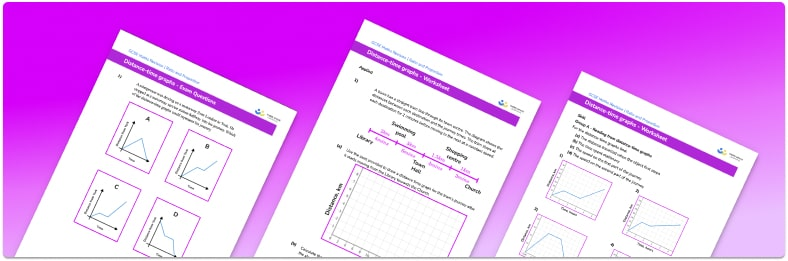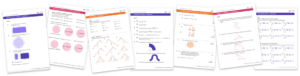# Distance Time Graph Worksheet• Section 1 of the distance time graph worksheet contains 20+ skills-based distance time graph practice questions, in 3 groups to support differentiation
• Section 2 contains 4 applied distance time graph questions with a mix of worded problems and deeper problem solving questions
• Section 3 contains 4 foundation and higher level GCSE exam style distance time graph questions
• Answers and a mark scheme for all distance time graph teaching resources are provided
• Questions follow variation theory with plenty of opportunities for students to work independently at their own level
• All questions and resource types are created by fully qualified expert secondary maths teachers

Suitable for GCSE maths revision for AQA, OCR and Edexcel exam boards

• This field is for validation purposes and should be left unchanged.

You can unsubscribe at any time (each email we send will contain an easy way to unsubscribe). To find out more about how we use your data, see our privacy policy.

### Distance time graph at a glance

A distance time graph shows how the distance of an object from a given point varies over time. On any distance time graph, a positive gradient indicates the object is moving away from the point and a negative gradient indicates the object is moving toward the point.

If the object is travelling at a constant speed, this will be shown as a straight line on the graph. A horizontal line represents a period of time when the object is stationary.

We can draw a distance time graph given some key information about a journey. We may need to use the formula for speed calculations, text{Speed} = text{Distance} div text{Time} to calculate unknown values.

When interpreting distance time graphs, the journey can be described one section of the graph at a time. It is important to remember that the total distance travelled must include all distances travelled in both directions and that the average speed of the object is calculated by dividing the total distance travelled by the total time of the journey.

Looking forward, students can then progress to additional rate of change worksheets and other ratio and proportion worksheets, for example a ratio worksheet or a simplifying and equivalent ratios worksheet.For more teaching and learning support on Ratio and Proportion our GCSE maths lessons provide step by step support for all GCSE maths concepts.

## Do you have KS4 students who need more focused attention to succeed at GCSE?There will be students in your class who require individual attention to help them succeed in their maths GCSEs. In a class of 30, it’s not always easy to provide.

Help your students feel confident with exam-style questions and the strategies they’ll need to answer them correctly with our dedicated GCSE maths revision programme.

Lessons are selected to provide support where each student needs it most, and specially-trained GCSE maths tutors adapt the pitch and pace of each lesson. This ensures a personalised revision programme that raises grades and boosts confidence.

Find out more